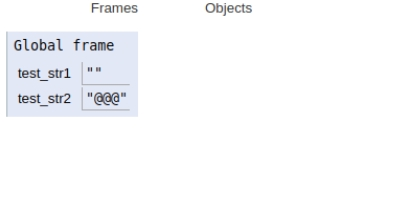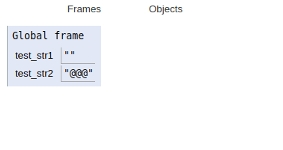# Python program to check if the string is empty or not

In this article, we will learn about the solution and approach to solve the given problem statement.

## Problem statement

Given a string input, we need to check if the string is empty or not.

Python strings are immutable in nature so while performing any operations care must be taken while handling strings.

Here we will discuss two approaches to solve the above problem statement −

• Using len() method.
• Using equality operator.

## Example

Live Demo

test_str1 = ""
test_str2 = "@@@"
if(len(test_str2) == len(test_str1)):
print ("Yes")
else :
print ("No")

## Output

No

All variables and functions are declared in global scope as shown in the figure below.## Example

Live Demo

test_str1 = ""
test_str2 = "@@@"
if(test_str2 == test_str1):
print ("Yes")
else :
print ("No")

## Output

No

All variables and functions are declared in global scope as shown in the figure below.## Conclusion

In this article, we learnt about the approach to find whether a string is a number pangram or not